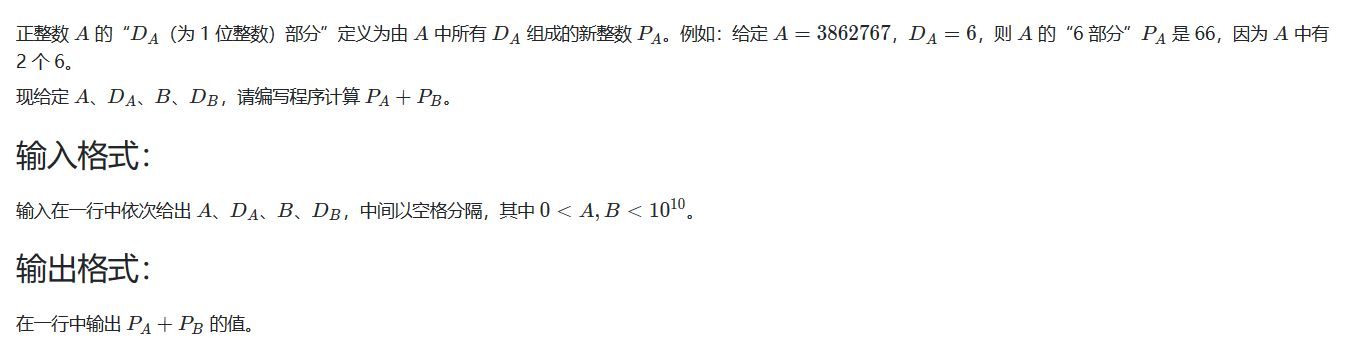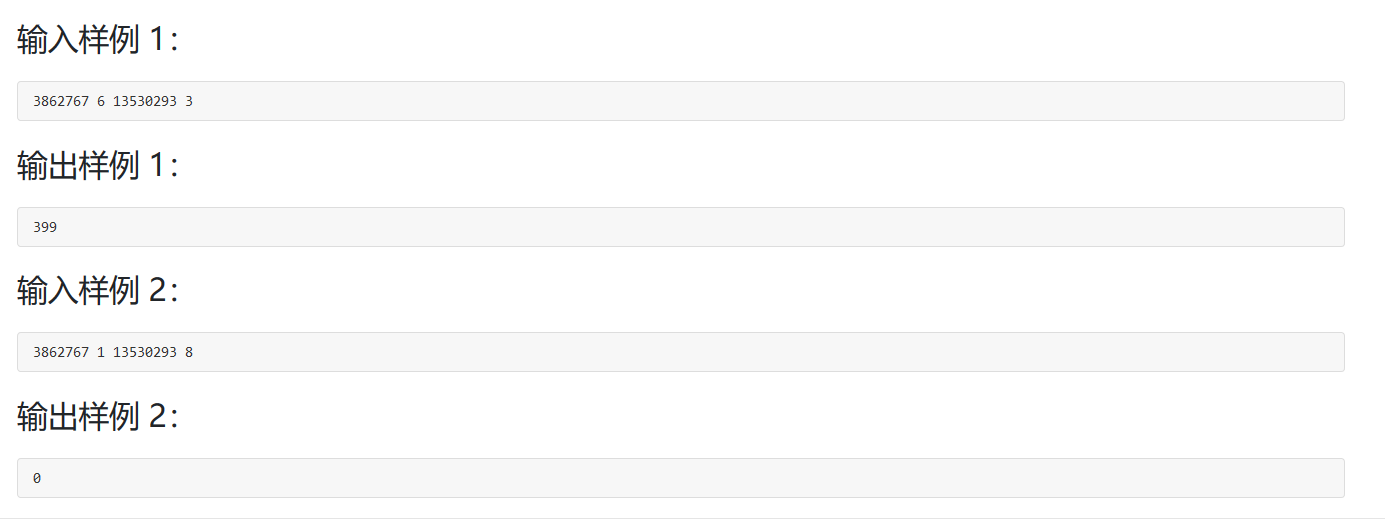# 浙江大学PAT (Basic Level) Practice （中文）1016部分A+BJAVA实现代码及分析

2019-03-21 22:34 148 查看

# 1016

## 部分A+B因为输入的数范围比较大，所以我个人的思路1是将输入的两个正整数以字符串转字符数组的格式输入并保存，然后根据输入的P的值进行字符比较得出相同字符的个数，然后将Pa和Pb的值计算并相加之后进行输出。

```import java.util.Scanner;

public class Main {

public static void main(String[] args)
{
Scanner input=new Scanner(System.in);
String a,b;
int da,db;
a=input.next();
da=input.nextInt();
b=input.next();
db=input.nextInt();
cal(a,b,da,db);
}
public static void cal(String a,String b,int da,int db)
{
char ca[]=a.toCharArray();
char cb[]=b.toCharArray();
int ia=0,ib=0;
char cda=(char) (da+48);
char cdb=(char)(db+48);
for(char item:ca)
{
if(item==cda)
{
ia++;
}
}
for(char item:cb)
{
if(item==cdb)
{
ib++;
}
}
int ta=0,tb=0;
for(;ia>0;ia--)
{
ta+=Math.pow(10, ia-1)*da;
}
for(;ib>0;ib--)
{
tb+=Math.pow(10, ib-1)*db;
}
System.out.println(ta+tb);
}
}```